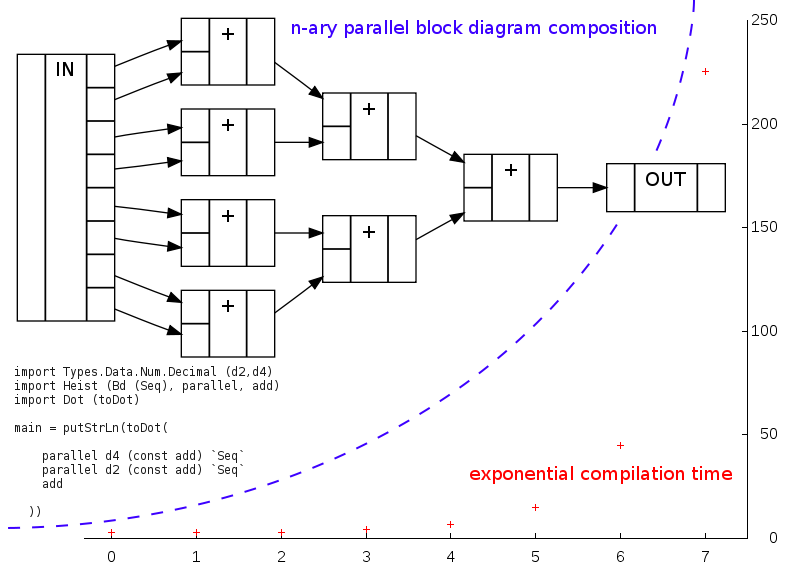# mathr / blog / #

## Heist: busted!FAuSt has a nifty n-ary block diagram parallel composition operator, in which you give it a count and a function from numbers to block diagrams. Something with the same meaning as:

parallel n f = foldr1 Par (map f [0..(n-1)])

If only life were so simple: that won't compile, because of my decision in Heist to express the type system of block diagram algebra within Haskell's type system. So after about 4 hours of head scratching, I finally figured out how to generalize from this:

par0 f = empty
par1 f = par0 f Par f 0
par2 f = par1 f Par f 1
par3 f = par2 f Par f 2
par4 f = par3 f Par f 3
...

to the point where this compiles successfully:

import Types.Data.Num.Decimal (d2, d4)
import Heist (Bd (Seq), parallel, add)
import Dot (toDot)

main = putStrLn(toDot(

parallel d4 (const add) Seq
parallel d2 (const add) Seq
))
svn co https://code.goto10.org/svn/maximus/2009/heist heist